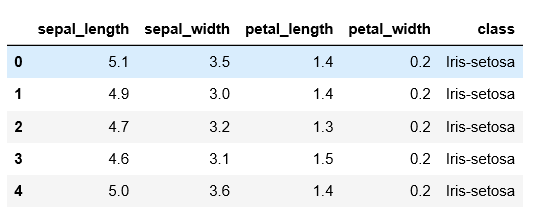top of page

# Data Manipulation With Pandas Library

Pandas is a fast, powerful, flexible, and easy-to-use open-source data analysis and manipulation tool, built on top of the Python programming language.

To install the pandas library

`pip install pandas`

We need to import the pandas library before using it.

`import pandas as pd`

`df = pd.read_csv('iris.csv')`

To explore data read we can use the .head or .tail method

`df.head()`.head() gives the top five rows of the dataframe. We can input the number of rows to .head() that we would like to read. Similarly, we can read the last five rows of the dataframe using the .tail method. For example, df.head(10) reads the first 10 lines of rows and df.tail(10) reads 10 rows from the last rows.

To read the unique values of the column

`df['sepal_length'].unique()`

All the unique values of the sepal_length column are printed.

Sorting the column

Values of the column can be sorted in ascending as well as descending column. The line below sorts the value of the sepal_length column in ascending order.

`data.sort_values('sepal_length',ascending=True)`

Subsetting data

We can subset the data frame also. The below subset the rows only if the class of the flower is iris-setosa with sepal_length greater than 5.

`data[(data['class']=='Iris-setosa') & (data['sepal_length']>5)]`

Check value contains in the column or nor using .isin

The .isin is used to check the value contains in the column or not. To use .isin the column must be categorical data. The code below gives the rows with 'Iris-virginica' value of categorical column class.

`data['class'].isin(['Iris-virginica'])`

Applying a statistical function to a column value

There are different types of statistical functions in the pandas library like mean, median, mode, standard deviation, etc. Amond them we have used maximum and sum functions which calculate the maximum value between the value of the sepal_length and the total sum of the values of the sepal_width.

```data['sepal_length'].max()
data['sepal_width'].sum()```

Aggregate function

We can apply a function to a single column or multiple columns using the .agg() function.

`data[['sepal_length','sepal_width','petal_length','petal_width']].agg(['min'])`

In the above line,we have use a minimum function to calculate a minimum value for column name 'sepal_length','sepal_width' ,'petal_length' and 'petal_width' columns.

We can apply a user-defined function to a single column or multiple columns also. From the example below, we have created a function named as test that takes an argument col. The test function calculates the maximum value and minimum value of the column passed to the test function. The test function is applied to all columns defined inside the square bracket of data. That means the test function calculates the maximum and minimum value of all of the columns sepal_length, sepal_width, petal_length, and petal_width.

```def test(col):
return col.max(),col.min()

data[['sepal_length','sepal_width','petal_length','petal_width']].agg(test)```

GroupBy

Pandas dataframe.groupby() function is used to split the data into groups. The dataframe data is grouped with respect to each value of the column class and count value of sepal_length for each class type.

`data.groupby(['class'])['sepal_length'].count()`

Pivot Table

The pivot_table() function is used to create a spreadsheet-style pivot table as a DataFrame.A simple example of the pivot_table is added to the snippet of the code. Count a sepal_length value for each individual value of the class column.

`data.pivot_table(values='sepal_length',index='class',aggfunc='count') `

Slicing the dataframe

We can select a part of dataframe using the .iloc and .loc methods. .loc is label-based, which means that we have to specify the name of the rows and columns that we need to filter out. On the other hand, iloc is integer index-based. So here, we have to specify rows and columns by their integer index.

`data.iloc[0:10,0:3]`

0:10 reads the first 10 ten rows and 0:3 reads 3 columns from the left side.

The command below reads a row only with value='Iris-setosa' and column name from 'sepal_length' to column name 'petal_length'.

`data.loc['Iris-setosa','sepal_length':'petal_length']`